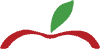Select Page

## MATH TUTORS

### Math Tutors With An Edge

Math can be challenging for a lot of students. It can be a source of anxiety and frustration. Our math tutors are skilled and teach concept in ways that will lead our students to success in math. Our one-on-one, customized math tutoring makes math interesting and fun by using games and real-life examples. As a result,  our students are presented with a clear system,  motivating them to master essential concepts.  Tutors on Call has math tutors who specialize in elementary school, high school, or university level mathematics.  We  offer the option of in-home or online math tutoring.### ELEMENTARY SCHOOL MATH TUTORS#### Tutor's Qualifications

Students in grades K – 7 would work with a school teacher or university degree specialist who understands the provincial math curriculum.#### Math Homework Help

Assignments are explained and any gaps can be improved upon by completing practice questions together.#### Filling-In Learning Gaps

Determine where these gaps are, reteach the concepts, assign homework, and test for understanding.#### Math Enrichment

Teach new concepts, provide more challenging questions, preview upcoming material, and introduce next year’s curriculum.#### Homeschooling Support

Work with the student to master the grade-level learning objectives while being a valuable resource and support to the family.

### HIGH SCHOOL MATH TUTORS#### Tutor's Qualifications

University degree in math or science and at least two years of tutoring experience in high school math.  Familiar with the provincial high school math learning outcomes.#### Math Homework Help & Test Preparation

Assignments and practice tests can be worked on together.#### Schooling Support

Help keep the student accountable and organized.  Guide students through the completion of assignments.#### Math Curriculum Review

Review last year’s curriculum to fill-in learning gaps and better prepare the student for this year’s course content.#### Our High School Math Courses

Math, Pre-Calculus, Calculus, Algebra, Functions, Advanced Functions, Statistics.  Full list of math curriculum

### UNIVERSITY MATH TUTORS#### Tutor's Qualifications

University degree with a background in math or science and several years of experience tutoring university math. Most of our university math tutors have a Ph.D. or master’s degree in maths or sciences.#### Math Homework Support

Tutor#### Math Test Preparation

Review the course material and complete practice tests together.#### Teach Math Content

Teach the material when the university instructor’s availability is limited.#### Our University Math Courses

Mathematical Literacy, Algebra, Calculus, Trigonometry, Geometry, Differential Equations, Statistics, and Engineering.

## MATH CURRICULUM

##### British Columbia

Gr. 8 Math, Gr. 9 Math, Gr. 10 Math Foundations, Gr. 10 Pre-Calculus, Gr. 11 Math Foundations, Gr. 11 Pre-Calculus, Gr. 12 Math Foundations, Gr. 12 Pre-Calculus, Gr. 12 Calculus, and Gr. 12 Statistics.

##### Alberta & NW Territories
Math 8, Math 9, Math 10C, Math 10-3, Math 20-1, Math 20-2, Math 20-3, Math 30-1, Math 30-2, and Math 30-3.

Math 8, Math 9, Foundations in Math and Pre-Calculus 10, Foundations in Math 20, Pre-Calculus 20, Foundations in Math 30, and Pre-Calculus 30.

##### Manitoba
Gr. 8 Math, Gr. 9 Math, Gr. 10 Essential Math, Gr. 10 Introduction to Applied and Pre-Calculus, Gr. 11 Applied Math, Gr. 11 Essential Math, Gr. 11 Pre-Calculus, Gr. 12 Applied Math, Gr. 12 Essential Math and Gr. 12 Pre-Calculus.
##### Ontario
Math 8, Principles of Math 9 (MPM1D,) Foundations of Math 9 (MFM1P,) Principles of Math 10 (MPM2D,) Foundations of Math 10 (MFM2P,) Gr. 11 Functions (MCR3U,) Gr. 11 Functions and Applications (MCF3M,) Gr. 11 Foundations (MBF3C,) Advanced Functions Gr. 12 (MHF4U,) Calculus and Vectors Gr. 12 (MCV4U,) Gr. 12 Data Management (MDM4U) and Gr. 12 Foundations.
##### New Brunswick

Math 8 and Math 9; Geometry, Measurements and Finances Gr. 10; Numbers and Relations and Functions Gr. 10, Foundations of Math 110, Pre-Calculus 110, Foundations of Math 120, Pre-Calculus A 120, Pre-Calculus B 120 and Calculus 120.

##### Nova Scotia

Math 8, Math 9, Math 10 Essentials, Math 10 Academic, Math 11 Essentials, Math 11 Academic, Math 11 Pre-Calculus, Math 12 Essentials, Math 12 Academic, Math 12 Pre-Calculus, and Math 12 Calculus.

##### Newfoundland

Math 8, Math 9, Math 1201, Applied Math 1202, Advanced Math 2200, Academic Math 2201, Applied Math 2202, Advanced Math 3200, Academic Math 3201, Applied Math 3202, and Calculus 3208.

##### Yukon

Gr. 8 Math, Gr. 9 Math, Foundations of Math and Pre-Calculus Gr. 10, Foundations of Math Gr. 11, Pre-Calculus Gr. 11, Foundations of Math Gr. 12 and Pre-Calculus Gr. 12.

## Get In Touch

Best Time to Reach You

Where did you hear about us?

5 + 9 =